# A Note on Bursting Pressure

1 / 8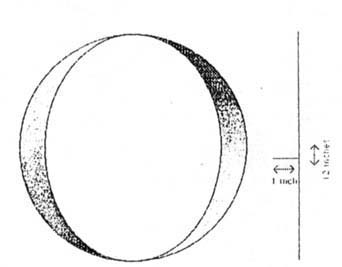Figure 1
2 / 8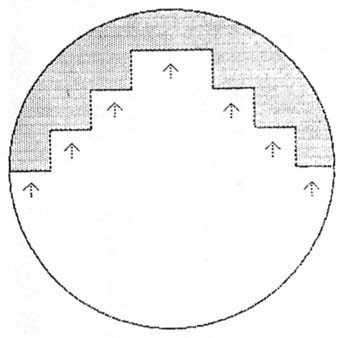Figure 2
3 / 8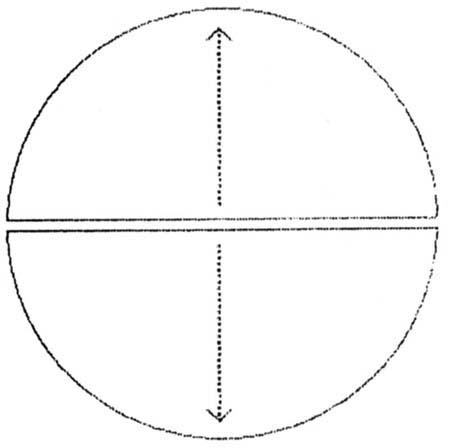Figure 3
4 / 8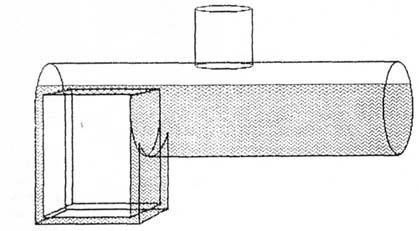Figure 4
5 / 8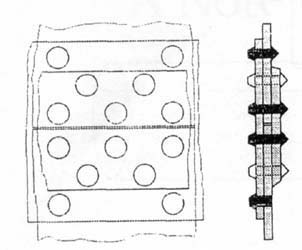Figure 5
6 / 8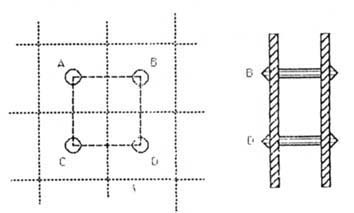Figure 6
7 / 8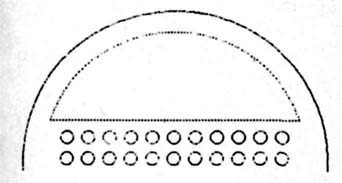Figure 7
8 / 8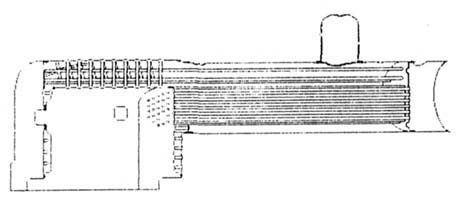Figure 8

735 Riddle Road Cincinnati, Ohio 45220

The internal combustion generation hardly conceives that, not so
long also, the topic of bursting pressure inspired raging debate.
In the steam era, when boilers proliferated, tempers, like water,
simmered on that subject. Knowledge of bursting pressure and the
closely related concepts of working pressure and factors of safety
mattered not only to designers of boilers and engines but also to
investigators at explosion sites, insurance companies, implement
dealers, and engineers. Equations for finding bursting pressure and
working pressure abounded. Most formulas did not differ so much in
substance as in degree of refinement; however, misunderstandings
provoked controversy between one school of thought and another. In
fact, to comprehend bursting pressure did require uncommon
concentration. Descriptions in reputable mechanical engineering
manuals and science textbooks from the late nineteenth and early
twentieth centuries helped to unravel the mysteries of bursting
pressure.

in 1897, asked engineering students to imagine a ring like that
depicted in Figure 1. If the ring were twelve inches in diameter
and one inch wide (or, to be more exact, slong), and if it could
contain an internal pressure of no more than one-hundred pounds to
the square inch, the force tending to burst the ring would be 12 x
1 x 100, or 1,200 pounds. That force, thus, consists of the product
found by multiplying the diameter, the length, and the pounds of
pressure per square inch.

Now, a reasonable human being might think that the
circumference, not the diameter, had better be used in the
equation. After all, is not the internal pressure pushing against
the whole ring, not merely an invisible diameter? Figure 2,
however, depicts the physical law that pressure in any direction is
effective on only those surfaces at right angles to that direction.
Symbolized by the arrows, the pressure pushes against the ring and
is resolved into the horizontal lines shown in the diagram. When
placed end to end, these horizontal lines add up to a diameter.

A reasonable person might also consider it logical to assume
that, if the pressure is, in effect, pulling a diameter in one
direction, then an equal pressure must be pulling a diameter in the
opposite direction. Figure 3 depicts such thinking. If this diagram
represents the ring discussed earlier, then one arrow signifies a
pressure of 1,200 pounds in one direction, and the other arrow
indicates a pressure of 1,200 pounds in the opposite direction. A
person might conclude that the bursting pressure is twice 1,200
pounds, or 2,400 pounds. The twin stresses of 1,200 pounds, though,
were exerted against each other. As The Power Catechism points out,
if two equally-strong people pull to the extent of their strength
on opposite ends of a rope, the effect on the rope is the same as
when one of the people pulls on the rope with its opposite end
attached to a solid post. In the same way, one side of the ring
pulls against the other side, and the tension in the ring is the
force with which each side pulls not double the force. The bursting
pressure for the ring, therefore, remains 1,200 pounds.

So far, the ring in these examples has been considered to have
length (one inch) and diameter (twelve inches) but no thickness.
Suppose it were one quarter of an inch thick. Further, assume it
were made of boiler steel having a tensile strength of sixty
thousand pounds per square inch. A bar of such steel one square
inch in cross section breaks when a pull of sixty thousand pounds
is applied in the direction of the bar’s length. To tear the
steel ring into two halves requires that it be torn apart at two
points. Assume that, after the break, the area of each of these
points will measure exactly one quarter of an inch by one inch. The
area exposed by one of the breaks, then, will equal one-quarter of
a square inch. The sum of the two areas will be one half of a
square inch. To burst these two points apart will need a force
equal to half of sixty thousand pounds per square inch, or
thirty-thousand pounds. This force must be exerted on twelve square
inches (the diameter of the ring multiplied by its length of one
inch). The bursting pressure will be thirty thousand divided by
twelve, or 2,500 pounds per square inch. The formula for this
operation follows:

(Thickness x length x tensile strength x 2 breaks)/diameter x
length = bursting pressure

Inserting the data makes the equation look like this:

(1/4 x 1 x 60,000 p.s.i. x 2)/I2x1 = 2,500 pounds per square
inch

Because length appears in both the numerator and the
denominator, it can be omitted. Also, dividing a diameter in two
gives a radius; the 2 in the numerator no longer is needed when
‘radius’ is substituted for ‘diameter.’ The
simplified equation now assumes this form:

(Thickness x tensile strength)/radius = bursting pressure

So long as the thickness and the radius are expressed in inches,
this formula will yield the bursting pressure in pounds per square
inch of a cylinder. A careful examination of this formula shows
that, with each increase in the radius, a cylinder becomes weaker.
If the thickness of a cylinder were one inch and the tensile
strength were sixty-thousand pounds per square inch, a cylinder of
a two inch radius would burst at thirty-thousand p.s.i., whereas a
cylinder with a radius of four inches would burst at
fifteen-thousand p.s.i.

Now enters the factor of safety, which is the number by which
the bursting pressure is divided to calculate the allowable working
pressure. In the steam era, factors of safety differed from year to
year, from manufacturer to manufacturer, and from model to model of
boiler and engine. Like bursting pressure the concept of a factor
of safety led to confusion and arguments.

If a builder were to allow a factor of safety of six, that would
not mean that the bursting pressure of a cylinder made of
boilerplate could be divided by six to get the safe pressure at
which the boiler and engine might be worked. A boiler takes a shape
far more complicated than that of a simple cylinder and has seams
which subtract from the strength of the plate itself. A glance at
Figure 4 will suffice to show how complex is the shape of the space
occupied by water and steam in a locomotive styled boiler. (The
schematic diagram of a boiler in Figure 4 is not intended to
resemble that of a particular builder and does not include such
parts as the tubes, the fire door, the grates, the smoke box, and
the smokestack.) The gray areas reveal how water flows beyond the
cylindrical form to cover the top, sides, and in this case
bottom of the firebox. Above the level of the water, steam fills
the space including the dome. Not shown are the riveted seams,
which hold together all of these shapes, the braces, which support
flat surfaces at various angles, and the numerous stay bolts, which
lend rigidity to the overall structure. The equation for the
bursting pressure of a simple cylinder cannot serve to indicate
what would be an appropriate factor of safety or the best working
pressure for a locomotive boiler. Nor does the cylinder formula
apply to a return flue boiler, wherein the large central flue
effectively changes the shape to that of a cylinder within a
cylinder.

Engineers in the design department of a boiler manufacturer had
to wield numerous theories and equations. In their efforts to find
the most accurate means of determining bursting pressure,
authorities disagreed. After all, their task was complicated. They
had to calculate precisely the relative strengths of various forms
of lap seam boilers and of butt strap boilers. Knowing that the
strongest joint results when the tensile strength of the sheet
between the rivet holes equals the shearing strength of the rivets,
engineers invented several configurations of seams, from lap seams
with one, two, or three rows of rivets to but strap joints with one
or two straps and with as many as six rows of rivets (see Figure 5
for front and side views of a butt strap joint). The Power
Catechism cautions that any seam constitutes a delicate balance:
‘If you give the rivets more pitch in order to increase the
sheet section, you lessen the number of rivets, increase the stress
on each, and the joint will fail by shearing. If  you put in
more rivets, you reduce the section of plate between them, and the
plate will pull apart. You can only gain strength in one factor by
sacrificing strength in the other, and the greatest strength will
obviously be when both are equal’ (41). To determine the proper
pitch, or distance between the centers of the rivets, designers of
boilers often used a five step formula: (A) multiply the number of
rows of rivets by the cross sectional area of the rivet hole; (B)
multiply this product by the shearing strength of the rivet; (C)
multiply the thickness of the plate by its tensile strength; (D)
divide the product obtained in step B by the products obtained in
step C, and (E) add the diameter of the rivet to the quotient
obtained after dividing. Engineers understood that even this
detailed method of deriving the pitch would not apply in every
situation.

Designers commonly used averages to simplify the mathematics
involved in building boilers. Such shortcuts included the
application of standard percentages of efficiency assigned to
certain configurations of seam used with specified thicknesses of
boilerplate and with particular riveting methods. For example, a
butt-joint with double straps, as compared to a seamless sheet of
boilerplate, might be assigned an efficiency of 87.5 percent. This
seam-efficiency percentage, then, could reduce the complexity of
calculating the bursting pressure and, hence, the factor of safety
and the working pressure. In his Steam Engine Guide (published in
1910 by the American Thresher-man Company in Madison, Wisconsin),
Phillip S. Rose uses such percentages in a rule for determining the
safe working pressure of a cylindrical boiler: ‘Multiply twice
the thickness of the boiler plate expressed in inches by the
ultimate tensile strength of the plate and this product by the
efficiency of the joint in percent. Divide this product by five
hundred times the diameter of the boiler, and the result is the
safe working pressure for a new boiler’ (29). Again, such an
equation applies only to a cylinder, not to the complicated shapes
of most boilers.

While figuring bursting pressures, mechanical engineers also had
to recognize that the stress on a longitudinal seam exceeds that on
roundabout seams. For example, if a tube sheet were solid, its
entire circumference would resist the boiler’s pressure per
square inch multiplied by the area, or the number of square inches
in that tube sheet. The area is found by this rule:

Diameter2 x .7854

The circumference is found by this method:

Diameter x 3.1416

For each inch of the roundabout seam, the resistance is found to
be in this proportion:

(Diameter2 x .7854)/Diameter Diameter x 3.1416 =
Diameter/4

The stress on each inch of the boiler’s length is in this
ratio: Diameter/2

The stress on a longitudinal seam, therefore, is twice that on a
roundabout seam, or, to put the same idea another way, a roundabout
seam is twice as strong as a longitudinal seam (all other matters
being equal). This observation, coupled with the fact that
cylinders having larger diameters will burst sooner than those with
smaller diameters, helps to explain why, with no increase in the
thickness of the boilerplate, larger and larger diameters of
boilers are weaker and weaker. The comparative weakness of the
longitudinal seam partly accounts for the need to thicken the plate
in accordance with each increase in the diameter of the boiler.

As if these calculations were not sufficiently daunting, Figure
4 plainly exhibits the difficulty of determining the forces at work
in the water and steam spaces surrounding the firebox. Designers
asked themselves what is the effect of the tube sheet holes which
accept the tubes, does this factor change once the tubes are
installed, what kind of supports and how many are needed to hold
the crown sheet, how should the firebox walls be strengthened, what
can be done to add durability to the upper portion of the front
tube sheet, how can the dome and waist be made strong, and similar
questions.

One rule of thumb for figuring the amount of stress permitted on
diagonal braces was to set the limit at six thousand pounds per
square inch of sectional area of a given brace. The allowance on
such a brace of a given diameter could be determined by multiplying
six-thousand by the square of the diameter and by .7854.

Next, to find the approximate stress which each stay bolt could
sustain, the engineer multiplied the pressure per square inch by
the area in square inches bounded by lines drawn between a given
stay bolt and its neighbors. Figure 6 shows such lines between stay
bolts, A, B, C, and D in the diagram at the left. To the right, a
side view of the subject reveals how the stay bolts support
parallel surfaces. If the distance between A and B equals eight
inches, and if the distance between B and D equals eight inches,
then the area is sixty-four square inches. Multiplying sixty four
by the pressure per square inch gives the approximate stress on any
of these stay bolts. For designers to determine the exact stress on
a given stay bolt demanded detailed knowledge of engineering and of
mathematics. To derive the diameter of a stay bolt, the designer
could begin by dividing the approximate stress by six-thousand (the
allowable working pressure on stay bolts). For example, if a stay
bolt were supporting sixty four square inches at 150 pounds per
square inch, the pressure would equal 9,600 pounds. Dividing this
figure by six thousand yields 1.6 of an inch. Dividing 1.6 by .7854
yields 2. The square root of 2 is 1.4. The diameter of the required
stay bolt, thus, is 1.4 of an inch. The best size of stay bolt to
use would round up to a diameter of one and a half inches.

Calculating the dynamic effects of pressure on a flat surface
required sophisticated computations. Rose hints at this complexity.
‘The tendency of pressure on the inside of any structure is to
form that structure into the shape of a sphere. If the walls of the
structure are flat they are subjected to a cross bending strain as
well as a tensile strain. A flat plate in a boiler must be treated
as a beam under a uniform load, and supported at the ends’
(30). Rose adds that a complete treatment of the problem is beyond
the scope of his book. He gives a rule for approximating the
allowable working pressure for plates seven sixteenths of an inch
and under. ‘ ‘Multiply the square of the thickness of the
plate in sixteenths of an inch, by 112 and divide this product by
the square of the distance from center to center of the stay
bolts.’ For thicker plates, 120 can be used in place of
112.

Regardless of the level of complication, figuring how to support
flat, rectangular surfaces was a snap, compared to determining how
to brace flat surfaces having curved perimeters, such as the
segment of the circle formed in the front tube sheet (or head)
above the tubes. Figure 7 depicts this area. Tables giving the area
of circular segments for a wide range of heights enabled designers
to simplify their work, but they still confronted a challenge in
knowing exactly where to place stay bolts for maximum effect in a
surface bounded by a curve. An even greater problem arose in
determining how to brace curving surfaces.

Figure 8 offers a schematic illustration of a Case 110
horsepower boiler. (Measurements are approximate.) Double thickness
of boilerplate in key areas, numerous stay bolts, and braces reveal
the genius of the designers. A comparison of Figure 4 and Figure 6
suggests the amount of stay s bolts and braces which mechanical
engineers deemed necessary for ensuring strength in a locomotive
styled boiler.

No wonder the topic of bursting pressure invoked controversy in
the steam era! The calculation of bursting pressure, appropriate
factors of safety, and working pressure challenged designing
engineers. In his Encyclopedia of American Steam Traction Engines,
Jack Norbeck writes, ‘One weakness of the Scheidler engine was
the support of the crown sheet. A lifetime engine man once remarked
that no one in his right mind would buy a Scheidler, since there
were always around the factory several old Scheidler boilers every
one of which had blown down in the crown sheet’ (247). Norbeck
explains that Reinhardt Scheidler did not support his crown sheets
in the customary way. Norbeck continues, ‘On the afternoon of
April 29, 1903, an engine under test in the factory blew down her
crown sheet, killing Scheidler instantly and injuring several
workmen near the engine.’ Mechanical engineers needed to
determine bursting pressure as accurately as possible. Then as now,
the difference between a good design and a great design can spell
the difference between death and life.

• Published on Nov 1, 1995
Farm Collector Magazine
Dedicated to the Preservation of Vintage Farm Equipment